# High School Physics : Understanding Scalar and Vector Quantities

## Example Questions

← Previous 1 3 4

### Example Question #1 : Understanding Scalar And Vector Quantities

Which of the following is a scalar quantity?

Acceleration

Displacement

All of these are scalar quantities

Force

Time

Time

Explanation:

The difference between a scalar and a vector is that a vector requires a direction. Scalar quantities have only magnitude; vector quantities have both magnitude and direction. Time is completely separated from direction; it is a scalar. It has only magnitude, no direction.

Force, displacement, and acceleration all occur with a designated direction.

Important distinctions to know:

Speed is a scalar, while velocity is a vector.

Distance is a scalar, while displacement is a vector.

Force and acceleration are vectors. Time is a scalar.

### Example Question #2 : Understanding Scalar And Vector Quantities

Which of the following is a vector quantity?

Displacement

Speed

Distance

All of these are vector quantities

Time

Displacement

Explanation:

A vector has both magnitude and direction, while a scalar has only magnitude. Ask yourself, "for which of these things is there a direction?" For displacement, we would say "50 meters NORTH," whereas with the others, we would say "50 meters," "20 seconds," or "30 miles per hour."

Important distinctions to know:

Speed is a scalar, while velocity is a vector.

Distance is a scalar, while displacement is a vector.

Force and acceleration are vectors. Time is a scalar.

### Example Question #3 : Understanding Scalar And Vector Quantities

Michael walks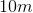north,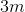west,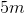south,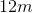east, and then stops to catch his breath. What is the magnitude of his displacement from his original point?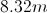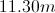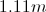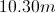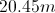Explanation:

Displacement is a vector quantity; the direction that Michael travels will be either positive or negative along an axis. We are being asked to solve for his position relative to his starting point, NOT for the distance he has walked.

First we need to find his total distance travelled along the y-axis. Let's say that all of his movement north is positive and south is negative.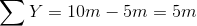. He moved a net of 5 meters to the north along the y-axis.

Now let's do the same for the x-axis, using positive for east and negative for west.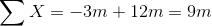. He moved a net of 9 meters to the east.

Now to find the resultant displacement, we use the Pythagorean Theorem. The net movement north will be perpendicular to the net movement east, forming a right triangle. Michael's position relative to his starting point will be the hypotenuse of this triangle.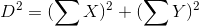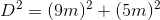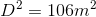Now take the square root of both sides.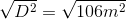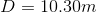Since the problem only asks for the magnitude of the displacement, we do not need to provide the direction.

### Example Question #4 : Understanding Scalar And Vector Quantities

Leslie walks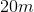north,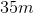east,north, and then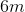west before stopping. What is her displacement from her original location?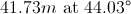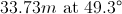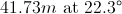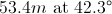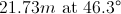Explanation:

Displacement is a vector quantity; it will have both magnitude and direction.

First we need to find his total distance travelled along the y-axis. Let's say that all of her movement north is positive and south is negative.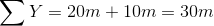. She moved a net of 30 meters to the north.

Now let's do the same for the x-axis, using positive for east and negative for west.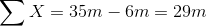. She moved a net of 29 meters to the east.

Now, to find the resultant displacement, we use the Pythagorean Theorem. Her net movement north will be perpendicular to her net movement east, forming a right triangle. Her location relative to her starting point will be the hypotenuse of the triangle.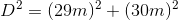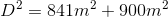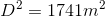Now take the square root of both sides.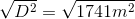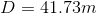Since we are solving for a vector, we also need to find the direction of this distance. We do this by solving for the angle of displacement.

To find the angle, we use the arctan of our directional displacements in the x- and y-axes. The tangent of the angle will be equal to the x-displacement over the y-displacement.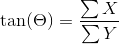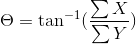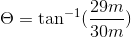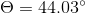Combining the magnitude and direction of our distance gives us the displacement: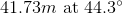.

### Example Question #5 : Understanding Scalar And Vector Quantities

Angie runs around a circular track for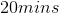. The track is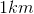and she runs at a rate of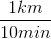. What is her total displacement?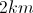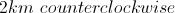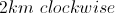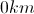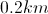Explanation:

Since she is running on a circular track, every time she makes a loop she has a total displacement of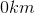. Remember, displacement take into account how far you've travelled, it only uses the total change in distance from where you start and where you stop. Using dimensional analysis, we can determine how many laps she runs in 20 minutes.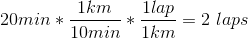After twenty minutes, she has made EXACTLY two loops around the track. That means she is starting and stopping in EXACTLY the same place. Her displacement would be, since there is no change between her starting position and her ending position.

### Example Question #6 : Understanding Scalar And Vector Quantities

Walter is washing windows on a large building. He starts by washing the window on the 4th floor, then down to the 3rd floor, then up to the 6th floor, then down to the 5th floor, then down to the 2nd floor, and finally he washes the 1st floor window. What is his total distance?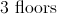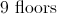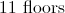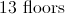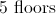Explanation:

Distance is a scalar quantity and will take into account only the number of floors travelled, regardless of the direction of movement.

Walter takes an incredibly complicated path to wash the windows on the building. When calculating distance, we add up all the movement he does, regardless of direction.

First, he travels down one floor (4th to 3rd).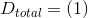Then he travels up three floors (3rd to 6th).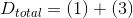Then he travels down one floor (6th to 5th), then down another three floors (5th to 2nd).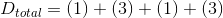Finally, he travels down one more floor (2nd to 1st).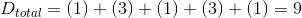In total, Walter travelled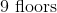.

### Example Question #7 : Understanding Scalar And Vector Quantities

Walter is washing windows on a large building. He starts by washing the window on the 4th floor. He then moves down to the 3rd floor, then up to the 6th floor, then down to the 5th floor, then down to the 2nd floor, and finally he washes the 1st floor window. What is his total displacement?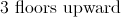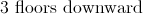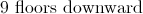Explanation:

Displacement is a vector relating the starting position to the ending position. Displacement does not take into account the route to arrive at the endpoint, and has both magnitude and direction.

In spite of taking a very complicated route to get there, Walter starts at the 4th floor and ends at the 1st floor.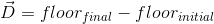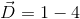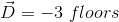Sine the result is negative, the displacement is 3 floors downward.

### Example Question #8 : Understanding Scalar And Vector Quantities

Ariel walksto the east,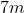to the west, and thento the east again. What is her total displacement?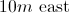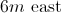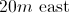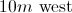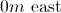Explanation:

Displacement is a vector quantity, with both magnitude and direction. Remember, displacement does not take into account the route traveled, only the difference between starting position and ending position.

All movement in this question occurs along the x-axis (east and west. We use positive for east and negative for west, since direction is important to measure displacement.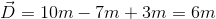This means that her total displacement was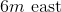.

### Example Question #1 : Understanding Scalar And Vector Quantities

Which of these is a vector quantity?

Temperature

Time

Speed

Distance

Acceleration

Acceleration

Explanation:

Scalar quantities give a magnitude, while vector quantities give a magnitude and a direction. The answer will be a measurement that must act in a given direction.

Distance is a measure of length, regardless of the direction. Displacement is the vector equivalent of distance.

Speed is a measure of rate, regardless of direction. Velocity is the vector equivalent of speed.

Temperature and time do not act in any direction and are purely scalar.

Acceleration must act in a given direction, and is a vector. An acceleration is described by both a magnitude and a direction of action.

### Example Question #1 : Understanding Scalar And Vector Quantities

Which of these is a scalar quantity?

Velocity

Force

Momentum

Displacement

Mass

Mass

Explanation:

Scalar quantities give a magnitude, while vector quantities give a magnitude and a direction. The answer will be a measurement that does not change, regardless of the direction of action.

Displacement is a measure of length in a given direction; distance is the scalar version of displacement.

Velocity is a measure of rate in a given direction; speed is the scalar version of velocity.

Force is a derivative of acceleration, and can only act in a given direction. There is no scalar equivalent of force. Similarly, momentum is a derivative of velocity and has no scalar equivalent.

Mass is a measure solely of magnitude, and requires no direction of action. Mass is a scalar quantity.

← Previous 1 3 4